Regularization is frequently used in statistics and machine learning to stabilize sensitive procedures in case of insufficient data.. It will be argued here that it is specifically of interest in pattern recognition applications if it can be related to invariants of the specific problem at hand. It is thereby a means to incorporate prior knowledge in the solution.

#### Regularization

The concept of regularization can be beautifully illustrated by one of the slides of prof. Kenji Fukumizu which is copied here. It shows a function approximation on the basis of a set of observations. There exist many smooth functions that exactly pass the given points. This solution, however, does not take into account possible measurement errors. Even when the observations are without noise, the function values outside the points may be very wrong.

It is better to smooth the solution. One way to do this is to add a penalty term, e.g. based on the length of the parameter vector that defines the function. The size of the trade-off parametershould either be chosen on the basis of experience, or optimized with a an independent set of observations or by cross-validation.

There are many other ways to realize a regularization. One is early stopping by limiting the number optimization steps. This prevents overtraining the set of function parameters. Another one is the addition of noise to the observations. This is especially helpful if the noise is different in every iteration (epoch) of the optimization procedure. The parameter updating will thereby in every cycle be directed into a different direction.

#### Invariants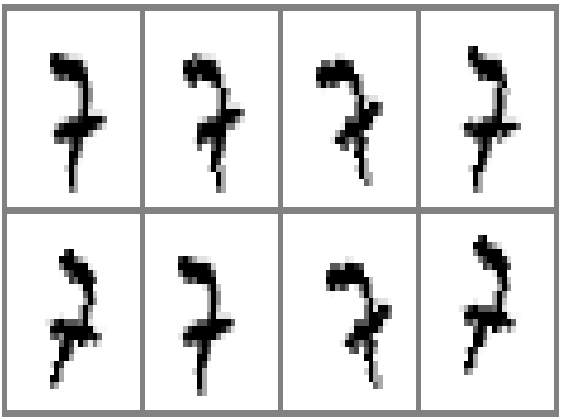The addition of noise to the data is equivalent to enlarging the dataset by additional observation. Arbitrary noise, however, is not very realistic. It might be more effective to enlarge the dataset by variations of observations that may be found in practice. These are the invariants. They construct a subspace in the feature space that is not informative for the class. For instance, all rotated and translated versions of the digit ‘7’ constitute other versions of a ‘7’ and will never transform into another digit. The generation of noise in the subspace of invariants is thereby similar to augmenting the dataset with other observation. This is thereby more effective than changing arbitrary pixels.

Regularization and invariants are related. The first is a technique mainly studied in machine learning. Knowledge about invariants may be obtained in analyzing the pattern recognition application and the chosen object representation. This may be helpful in defining an effective regularization.

#### Ridge regression

The equivalence of regularization and invariants may be illustrated by the use of ridge regression in the estimation of covariance matrices e.g. needed in the linear Fisher discriminant. If we have a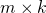data matrix forobjects represented in a-dimensional vector space, the covariance matrix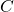of the underlying distribution can be estimated by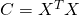in which we assume that the objects have zero mean (or are shifted to the origin before). This matrix needs to be inverted in the computation of a classifier. It will, however, be singular (having rank 0) in case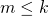, i.e. less objects than dimensions. One solution might be to add a term to the estimate: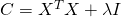in which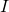is the identity matrix. For a sufficiently large, e.g.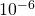the estimatedbecomes invertible. This is equivalent to adding a set of objects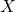identical to the original objects, but with additive noise with a variance related to. In many applications a some noise is equivalent to an invariant as it has no influence on the class membership of that object.

Sometimes it is known (or can be measured from) how large the feature correlations are at least. This holds for instance in images if pixels are used as features. In such a case the identity matrix may be replaced by the correlation matrix by winch more effectively the prior knowledge about invariants is used.

#### Conclusion

In summary, regularization is equivalent to extending the dataset with invariants. This may be done explicitly by constructing such objects, or implicitly by introducing mathematical terms in which knowledge about the invariants has been incorporated.

Filed under: OvertrainingRepresentation